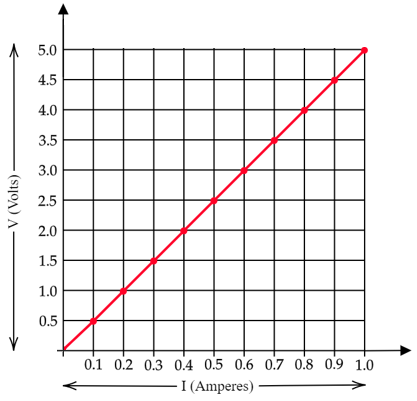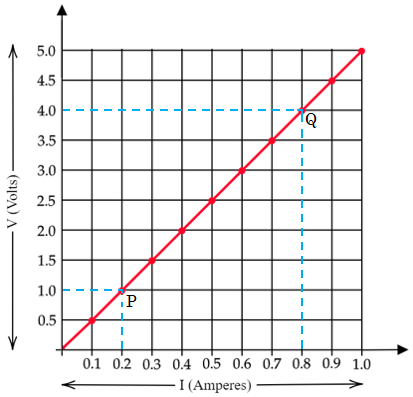# The values of current (I) flowing through a given resistor of resistance (R), for the corresponding values of potential difference (V) across the resistor are as given below :V (Volts)0.51.01.52.02.53.04.05.0I (Amperes)0.10.20.30.40.50.60.81.0Plot a graph between current (I) and potential difference (V) and determine the resistance (R) of the resistor.

The plot between current $(I)$ and potential difference $(V)$ is given as-Resistance of the resistor (R) = Slope of the above graph

Choose any two points P and Q on the graph,Resistnce of the resistor (R) = Slope of line $PQ=\frac{4-1}{0.8-02}=\frac{3}{0.6}=\frac{30}{6}=5\Omega$

Alternate Solution

We know that-

$R=\frac{\Delta V}{\Delta I}$

Where,

$R$ = Resistance

$V$ = Potential difference or Voltage

$I$ = Current

$\Delta$ = shows change

Putting the given values we get-

$R=\frac{3-2}{0.6-0.4}$

$R=\frac{1}{0.2}$

$R=\frac{10}{2}$

$R=5\Omega$

NOTE: For every point on the graph, the resistance will remain the same.

Updated on: 10-Oct-2022

108 Views